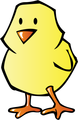#### You may also like### Kissing

Two perpendicular lines are tangential to two identical circles that touch. What is the largest circle that can be placed in between the two lines and the two circles and how would you construct it?### Good Approximations

Solve quadratic equations and use continued fractions to find rational approximations to irrational numbers.### Target Six

Show that x = 1 is a solution of the equation x^(3/2) - 8x^(-3/2) = 7 and find all other solutions.

# Bird-brained

##### Age 16 to 18Challenge Level

A mother bird has 2kg of mass available to produce her eggs. The chance of an egg surviving to hatch (which we will call p) is greater for heavier eggs.

This chance is defined by the following function:

p = 0       if individual egg mass (which we will call s) $\le{150}$g

p = $\frac{s-150}{k+s-150}$       if s > 150g, where k is a constant

Sketch a graph of this function. Can you describe how survival chance, p, is affected by egg mass, s? What assumptions have been made in applying this model?

How does the shape of the graph change as k varies? (Try thinking about what will happen if k is very big or very small)

if k = 0.4, what is the optimal size for each egg to be? Hence, how many eggs should the bird lay to produce the greatest expected number of chicks? What can you say about this number? If k was bigger, would the optimal number of eggs increase or decrease?

Given that a bird cannot actively choose how many eggs to produce and what mass each should be, do you think that birds in nature produce the optimal number of eggs? Why do you think this?